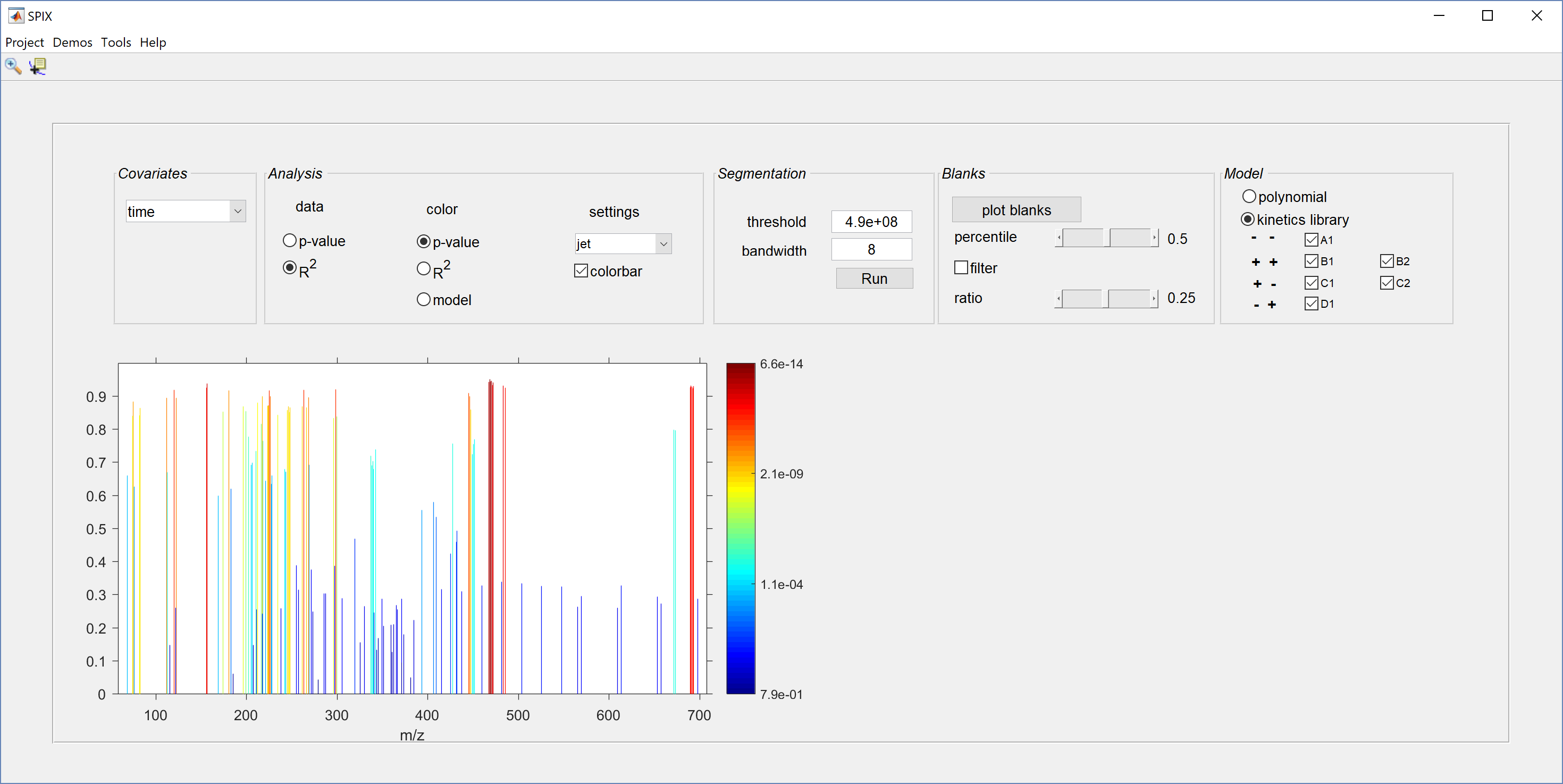# 1 Fitting kinetic models

Imagine that we are interested to see how the spectrum changes over time during some experiment.

For that, we first need to select time as covariate. We then try to describe by means of kinetic models how the intensity of each spike evolves over time.

For each species/\(m/z\), SPIX

• fits a kinetic model to the data,
• compute \(R^2\), i.e. the fraction of variance of the data explained by the model,
• compute the \(p\)-value of the test used for testing if the model reduces to a constant model (no trend). Then, a small \(p\)-value indicates that we are confident that the intensity of the spike, i.e. the amount of the associated species, changes over time.For each species, the ``best’’ kinetic model is selected among a library of 7 models with different shapes:

• models \(A_1\) and \(A_2\) decrease over time
• models \(B_1\) and \(B_2\) increase over time
• models \(C_1\) and \(C_2\) increase and then decrease
• models \(D_1\) decreases and then increases